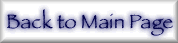## 9. Summary of uncertainties

### f2 and [µ/ρ]PE

Estimates of uncertainties are provided in Table 2. Relative uncertainties in f2 and [µ/ρ]PE are identical. Form factors are given in units of electrons per atom (e/atom). The accuracy of f2 in this work and C95 in a central x-ray region well away from edges is estimated as 1 %. This claim leads to significant discrepancies with other theory and experiment, which must be the subject of future investigation.

At low energies (especially below 200 eV) the uncertainty of C95 is very large and reaches 100 % to 200 %. This is due to the dominance of non-IPA interactions in this region. The best estimate of the accuracy of reported structure in this region (in C95) is given by comparison to an independent experimental or theoretical source, such as Henke et al. .

Accuracies below 1 keV reach a few percent or better in the absence of edge structure, and accuracies somewhat above edges are intermediate in estimated accuracy.

In the near-edge regions of direct concern to this work, the results of C95 should be replaced by the current tables in overlapping regions. Then the estimated uncertainty of f2 in the combined tabulation (for energies and edges above 1 keV) is 20 % to 30 % compared to a monatomic gas form factor (i.e., ignoring the effects of solid state structure and XAFS, for example). The presence of molecular or solid state interactions can lead to dramatic excursions from theory for a monatomic gas in these regions, which can exceed 50 % in extreme cases.

### Energies

In this near-edge region the location of the edge is critical to a general comparison, and this work uses the same experimental edge energies as C95. They are usually in good agreement with experiment (say to a few eV) and with those listed for ref. . However, there are exceptions to this as indicated in the plot for gallium, Z = 31, where discrepancies might reach 100 eV. Some of these variations are due to experimental calibration errors or to chemical shifts of up to 10 eV. Others are not clear at this point, and we have preferred to be consistent with C95 than to alter any of these.

### f1 - Zeff

The precision of f1 is dominated by that of f′, so any percentage uncertainty should be expressed in terms of this or of f1 - Z. However, below an edge the orbitals do not contribute to f2 and do not need to be computed for f2 except for near-edge discrete transitions. Hence the effective Z is reduced. However, as seen for Nd, Z = 60, and other elements, an error of f2 at an edge can significantly affect the value of f′ some order of magnitude below the edge. The typical ideal is represented by the results for krypton, Z = 36, where an error (or uncertainty) in f2 has lead to errors of some 50 % for f′ in the near-edge region, but has had no effect some factor of two in energy below the edge. Further, we find that at that point a factor of two in energy below the edge, the value of f1 correctly represents the number of effective oscillators at this energy - i.e., 30 rather than 36 (for forward scattering). An error of f2 at a lower energy would therefore be expected to have an effect on the region of the edge (within a factor of two) at the 50 % level, applied to f′ - 30.

C95 has discussed several types of error corresponding to 100 % to 200 % errors in this value near edges. While this work is free of those errors, any computation of f′ is extremely sensitive near edges to the sharpness of the discontinuity in f2. As such there will remain significant uncertainties in the near edge region, of perhaps 50 %. In any application of Re(f) away from forward scattering, the uncertainty in f0 computations (or in f0 - Z) may be 1 % to 5 % and may dominate over the uncertainty in the anomalous dispersion f′.

### frel

Because of the nature of the computation, the error in f1 at very high energies can be very small and much less than 0.1 e/atom within the current formalism. This may compare with previously discussed limitations at the 1-3 e/atom level of some sources . This is because f1 - Z becomes very small and indeed dominated by the relativistic correction frel and that associated uncertainty. We do not tabulate this uncertainty because the experimental evidence is usually limited by other uncertainties. An upper estimate for old sources of data is represented by the differences between the two values provided in the header of the tables for each element. This "H92 - 3/5CL" value is 1.09 e/atom for uranium or 0.002 e/atom for Z = 6 (i.e., 40 % of the dipole correction). However, we suggest as a lower estimate of uncertainty the difference between the results of Creagh ,  and Kissel . This reaches 19 % of the total relativistic correction for Z = 90, or 0.3 e/atom, or 1 % of the relativistic correction for Z = 6.

### [σ/ρ]coh+inc

The column for Rayleigh scattering and incoherent scattering is of no value for angle-dependent studies (this should be recomputed as discussed above). It is also of little value in experimental comparisons of aligned or misaligned Bragg-Laue scattering, except to estimate the magnitudes of these processes (again, see the discussion of uses of this tabulation). However, it allows us to compute a total attenuation coefficient [µ/ρ] in the tables, and to point out the relative significance of isolated atomic scattering for each element at various energies. This is the prime use to which this column should be put.

The accuracy of this computation for scattering varies, and also improves for higher energies, but we estimate it to lie at the 5 % level. This value is generally consistent with discrepancies from ref.  and ref.  claims to approach 1 % to 2 % in several regimes, but notes some particular deviations of 10 % for heavy elements at 100 keV, for example.